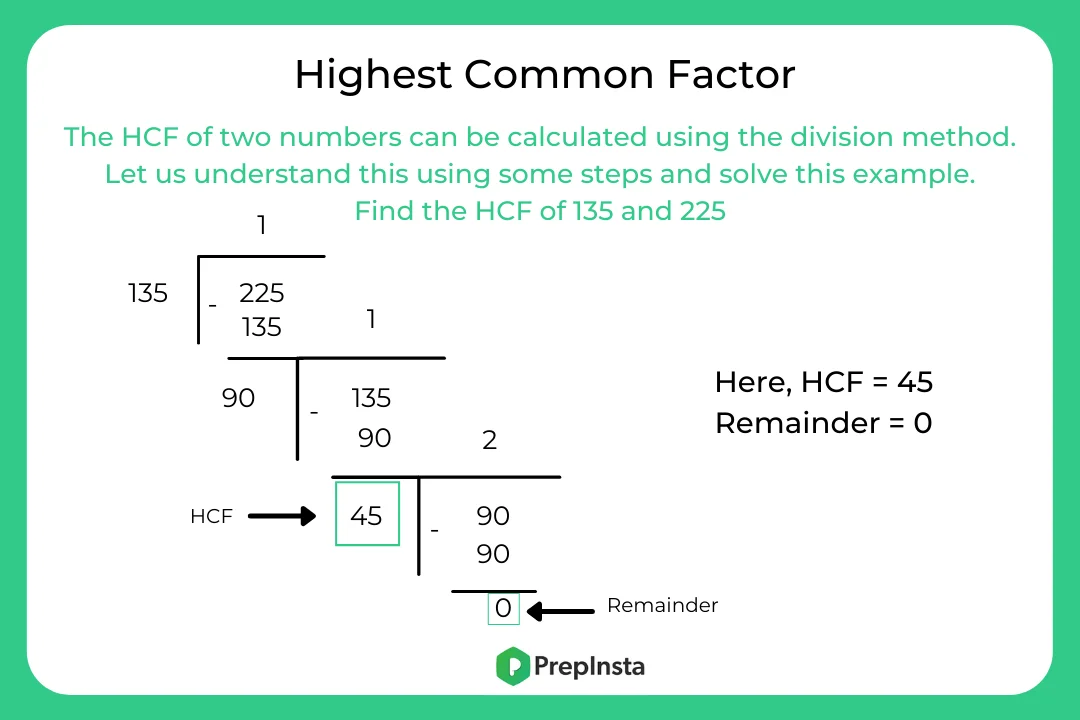# HCF Formulas

## Basic Concepts and Formula for HCF

On this page you will get the definition, methods to find HCF and formulas of HCF that are helpful in the preparation of HCF questions. HCF stands for Highest Common Factor. It is highest positive integer that divides two or more integers. That’s why it is also known as Greatest Common Divisor (GCD).### Definition

• HCF – The H.C.F of two or more than two numbers is the greatest numbers which divides each of them without any remainder. It also called Greatest Common Divisor (G.C.D.) or Greatest Common Measure (G.C.M.)

### How to Find HCF?

There are multiple ways to find the highest common factor or HCF of the given numbers. Irrespective of the method, the answer to the HCF of the numbers would always be the same. Here are 3 methods to calculate the HCF of two numbers:

• HCF by listing factors method
• HCF by prime factorization
• HCF by division method

### Formulas For HCF

• There are different HCF formulas that are used to determine the HCF of the given numbers. Let’s see what they are:
• LCM × HCF = Product of the Numbers. Suppose P and Q are two numbers, then – LCM (P & Q) × HCF (P & Q) = P × Q.
• HCF of co-prime numbers is always 1. Therefore, LCM of Co-prime Numbers = Product of the numbers
• Euclid’s Division Lemma is used to find the HCF of 2 numbers. Get two numbers a and b, such that a> b. Find a/b. i.e. Dividend = Divisor × Quotient + Remainder. If the remainder is 0 then the divisor is the HCF, else apply the lemma on b and r and keep dividing until the remainder is 0.
• To find the HCF of fractions, we use HCF of fractions = HCF of numerators/LCM of the denominators
• To find the greatest number that will exactly divide p, q, and r. Required number = HCF of p, q, and r
• To find the greatest number that will divide p, q, and r leaving remainders a, b and c respectively. Required number = HCF of (p -a), (q- b) and (r – c)
• HCF of any two or more numbers is never greater than any of the given numbers.### Question and Answer for HCF

Question : Find the Highest Common Factor of 55, 65 and 75.

A. 2
B. 3
C. 4
D. 5

Solution:
Given, three numbers as 55, 65 and 75.

We know, 55 = 5 × 11

65 = 5 × 13

75 = 5 × 15

From the above expression, we can say 5 is the only common factor for all three numbers.

Therefore, 5 is the HCF of 55, 65 and 75.

Question : 6 and 7 are two co-prime numbers. Using these numbers verify the HCF formula, LCM of Co-prime Numbers = Product of the numbers.

A. 45

B. 46

C. 42

D. 47

Solution: Let’s find the LCM and HCF of 6 and 7

6 = 1×2×3

7= 1×7

LCM = 1×2×3×7 = 42 HCF = 1

The HCF formula states that LCM of Co-prime Numbers = Product of the numbers

Product of the numbers = 6×7 = 42

Hence, LCM of Co-prime Numbers = Product of the numbers i.e. 42 = 42. Therefore, it is verified.

Question  : Find the HCF of 18/10, 12/15, 24/20, 30/5

A. 3/400

B. 4/500

C. 6/400

D. 8/400

Solution: According to the HCF formula, HCF = HCF of numerator/LCM of the denominator.

So finding the HCF of the numerators:

18 = 2×3×3

12 = 2×2×3

24 = 2×2×2×3

30 = 2×5 × 3

Therefore, the HCF of 9, 8, 12, 18 = 3

10 = 1×2×5

15 = 1×3×5

20 = 1×2×4×5

5 = 1×5

Therefore, the LCM of 10, 15, 20, 5 = 2×5×2×4×5 = 400

HCF = HCF of numerators/LCM of the denominators

HCF = 3/400

Therefore, the HCF of 9/10, 6/15, 12/20, 18/5 is 3/400.

Question : Rakesh joshi has calculated the H.C.F. and L.C.M. of two 2- digit numbers as16 and 480 respectively. The numbers are :

A. 40, 48
B. 60, 72
C. 64, 80
D. 80, 96

Ans: 80, 96
H.C.F. of the two 2-digit numbers= 16
Hence, the numbers can be expressed as 16x and 16y, where x and y are prime to each other.
Now,
First number \times second number =$H.C.F. \times L.C.M.$
=> $16x \times 16y = 16 \times 480$
=> xy = $16 \times \frac{480}{16} \times 16= 30 ´$
The possible pairs of x and y, satisfying the condition xy = 30 are :
(3, 10), (5, 6), (1, 30), (2, 15)
So the numbers are (48,160) (80,96)

Question : The HCF of the two numbers is 29 & their sum is 174. What are the numbers?

A. 29 and 145
B. 30 and 150
C. 40 and 140
D. 65 and 130

Solution:

Let the two numbers be 29x and 29y.

Given, 29x + 29y = 174

29(x + y) = 174

x + y = 174/29 = 6

Since x and y are co-primes, therefore, possible combinations would be (1,5), (2,4), (3,3).

The only combination that follows the co-prime part is (1,5)

For (1,5): 29 x = 29 x 1 and 29 y = 29 (5) = 145

Therefore, the required numbers are 29 and 145.

### Related Banners

Get PrepInsta Prime & get Access to all 200+ courses offered by PrepInsta in One Subscription

## Get over 200+ course One Subscription

Courses like AI/ML, Cloud Computing, Ethical Hacking, C, C++, Java, Python, DSA (All Languages), Competitive Coding (All Languages), TCS, Infosys, Wipro, Amazon, DBMS, SQL and others